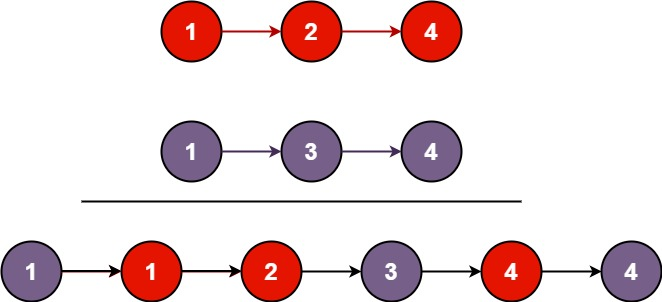Coding

# How to merge two sorted lists

0
(0)

Below we will try to solve a problem

Problem

Merge Two Sorted Lists

You are given the heads of two sorted linked lists `list1` and `list2`.

Merge the two lists in a one sorted list. The list should be made by splicing together the nodes of the first two lists.

Example 1:```Input: list1 = [1,2,4], list2 = [1,3,4]
Output: [1,1,2,3,4,4]
```

Example 2:

```Input: list1 = [], list2 = []
Output: []
```

Example 3:

```Input: list1 = [], list2 = 
Output: 
```

Constraints:

• The number of nodes in both lists is in the range `[0, 50]`.
• `-100 <= Node.val <= 100`
• Both `list1` and `list2` are sorted in non-decreasing order.

Solution

The way I approach solving this problem is using a two pointer approach. I have one pointer pointing to beginning of list1. And another pointer pointing to beginning of list 2. And then move these pointers in such a way that the smaller item of either list is added in result list, and corresponding pointer is moved to next element in that list. Once I reach the end of either list, I add the remaining elements of larger list to the result list and return that list.

ListNode class below represents typical LinkedList implementation in Java. Please refer to mergeTwoLists method for detailed implementation.

I would highly recommend you solve this problem by yourself before looking into the solution. One thing that I have realized while solving problems related to LinkedList is that they are very easy to come up with a pseudo solution with. But we sometimes miss moving to next pointers, or mess up in boundary cases which become hard to debug and find out where we might be committing those mistakes. In those cases, don’t shy away from coding and debugging this in your favorite IDE Eclipse or Idea.

``````public class ListNode {

int val;
ListNode next;
ListNode() {}
ListNode(int val) {
this.val = val;
}
ListNode(int val, ListNode next) {
this.val = val;
this.next = next;
}

@Override
public String toString() {
StringBuilder builder = new StringBuilder();
ListNode node = this;
while (node != null) {
builder.append(node.val).append("->");
node = node.next;
}
return builder.toString();
}
}

``````
``````public class TwoListMerger {

public static void main(String[] args) {
ListNode list1 = new ListNode(-9);
list1.next = new ListNode(3);

ListNode list2 = new ListNode(5);
list2.next = new ListNode(7);

ListNode listResult = new TwoListMerger().mergeTwoLists(list1, list2);
System.out.println(listResult);
}

public ListNode mergeTwoLists(ListNode list1, ListNode list2) {
ListNode c1 = list1;
ListNode c2 = list2;
ListNode result = new ListNode();
while (c1!=null && c2!=null) {
if (c1.val < c2.val) {
result.next = new ListNode(c1.val);
c1 = c1.next;
}
else {
result.next = new ListNode(c2.val);
c2 = c2.next;
}

result = result.next;
}

while (c1 != null) {
result.next = new ListNode(c1.val);
result = result.next;
c1 = c1.next;
}

while (c2 != null) {
result.next = new ListNode(c2.val);
c2 = c2.next;
}

}
}
``````

Click on a star to rate it!

Average rating 0 / 5. Vote count: 0

No votes so far! Be the first to rate this post.

As you found this post useful...